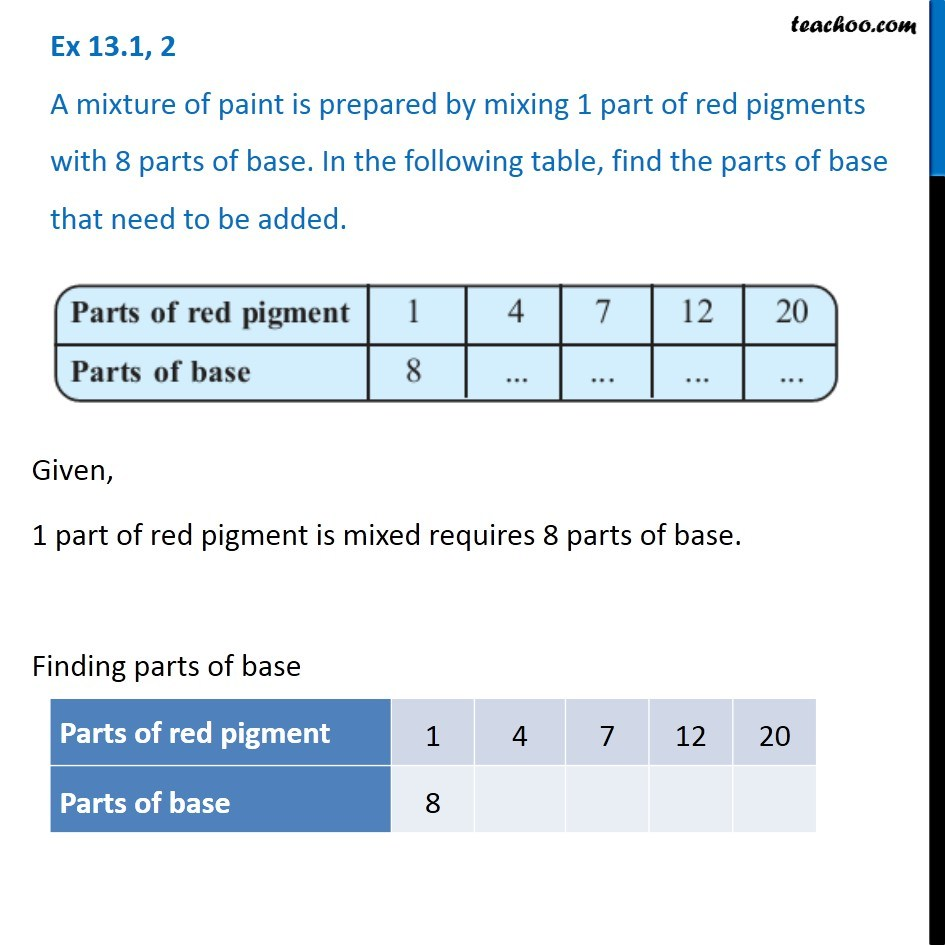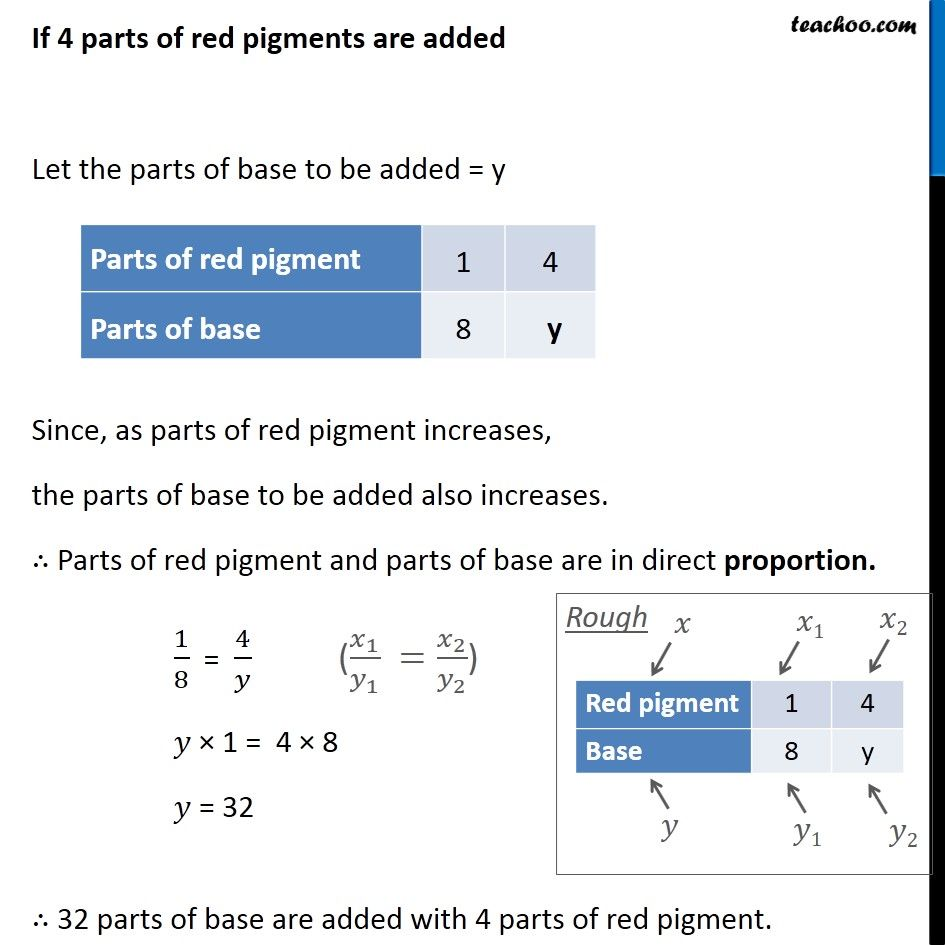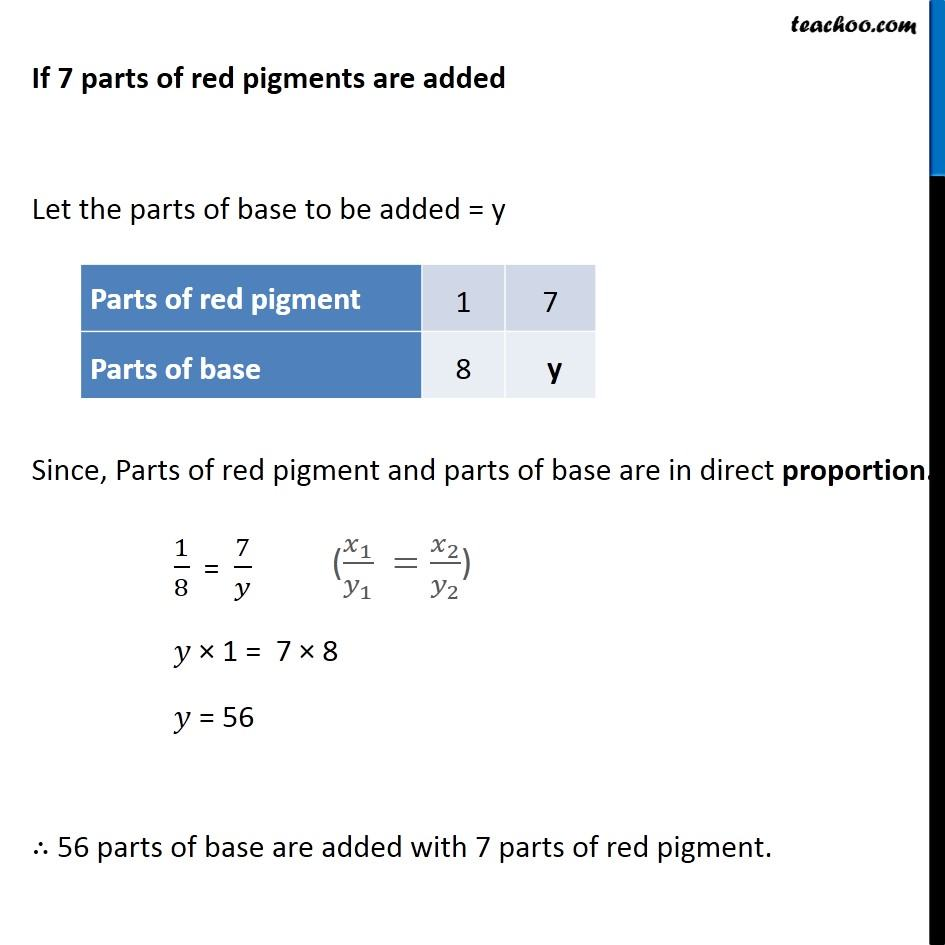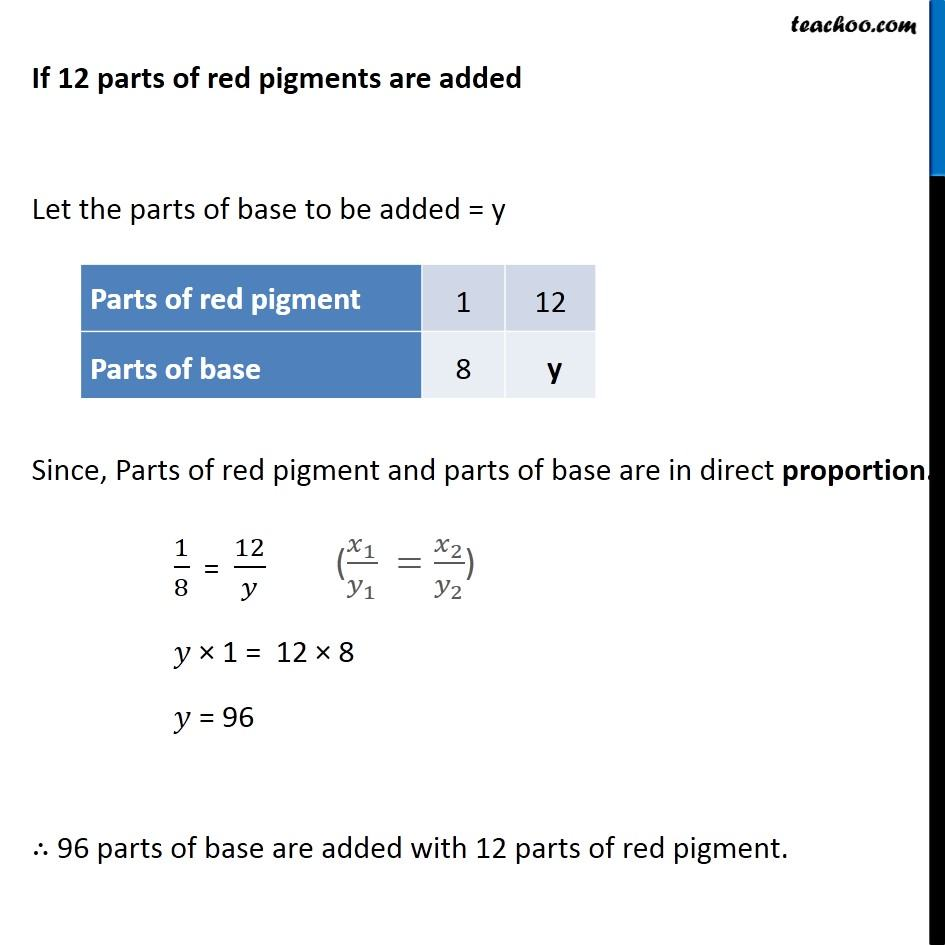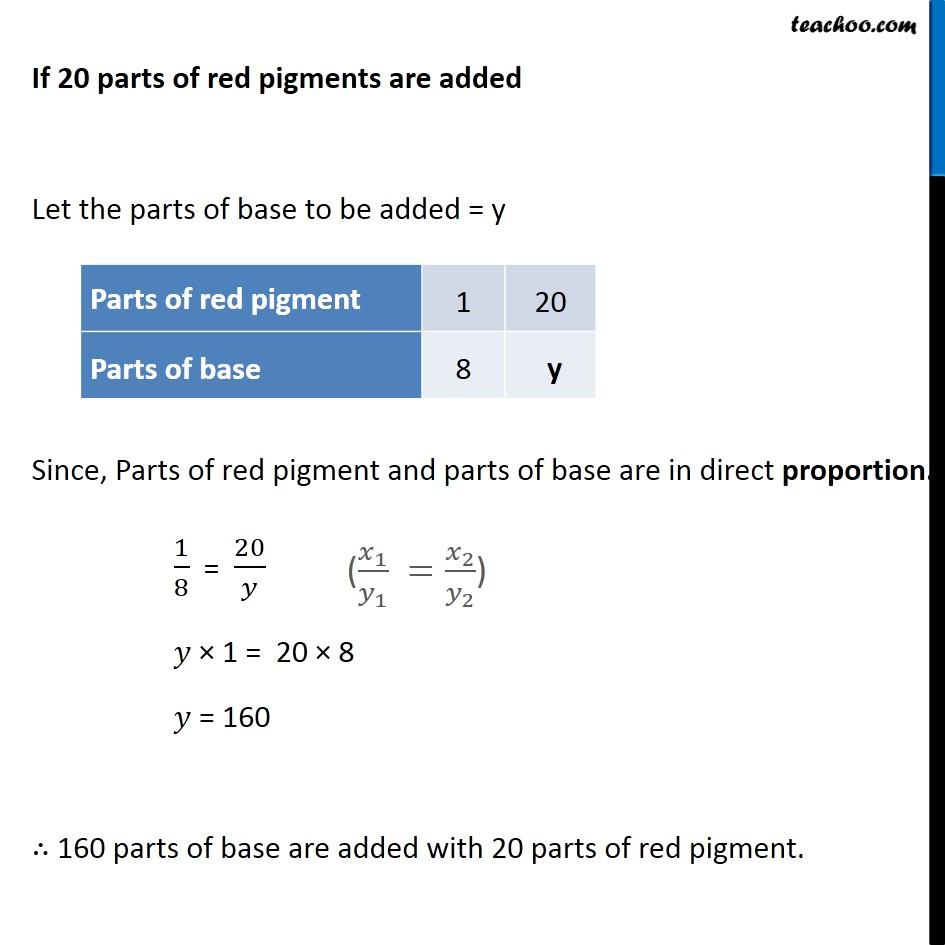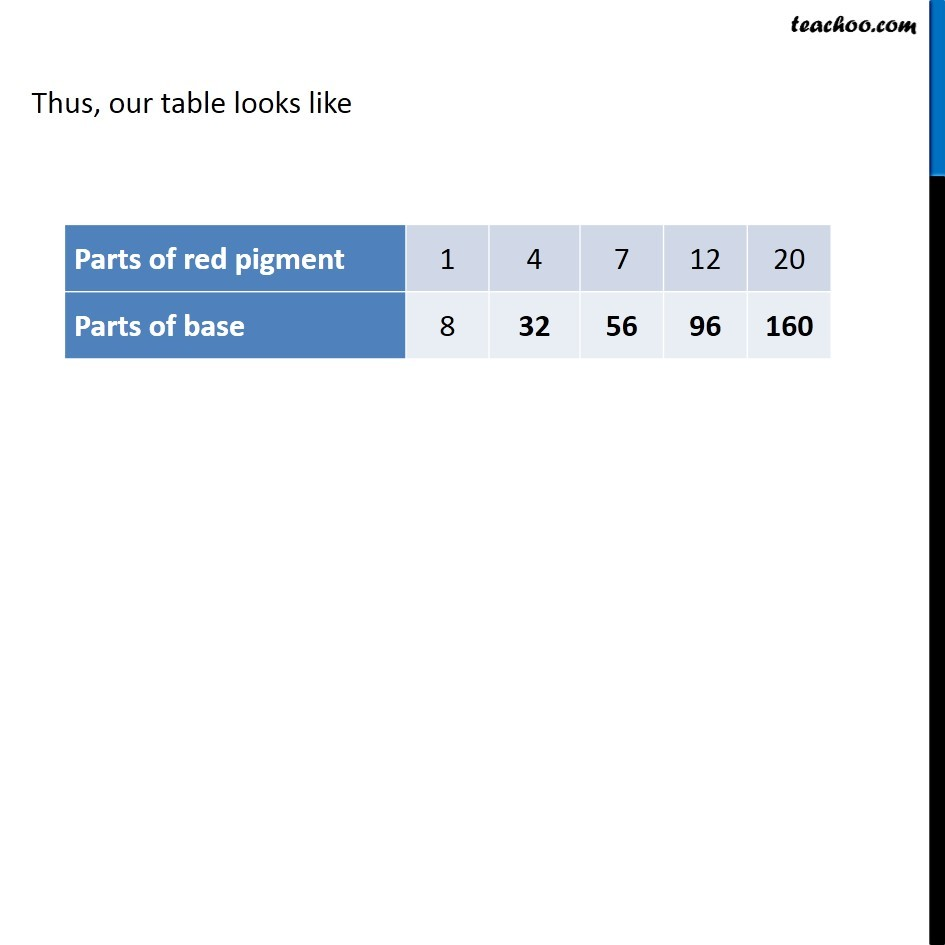1. Chapter 13 Class 8 Direct and Inverse Proportions
2. Serial order wise
3. Ex 13.1

Transcript

Ex 13.1, 2 A mixture of paint is prepared by mixing 1 part of red pigments with 8 parts of base. In the following table, find the parts of base that need to be added. Given, 1 part of red pigment is mixed requires 8 parts of base. Finding parts of base If 4 parts of red pigments are added Let the parts of base to be added = y Since, as parts of red pigment increases, the parts of base to be added also increases. ∴ Parts of red pigment and parts of base are in direct proportion. 1/8 = 4/𝑦 𝑦 × 1 = 4 × 8 𝑦 = 32 ∴ 32 parts of base are added with 4 parts of red pigment. If 7 parts of red pigments are added Let the parts of base to be added = y Since, Parts of red pigment and parts of base are in direct proportion. 1/8 = 7/𝑦 𝑦 × 1 = 7 × 8 𝑦 = 56 ∴ 56 parts of base are added with 7 parts of red pigment. If 12 parts of red pigments are added Let the parts of base to be added = y Since, Parts of red pigment and parts of base are in direct proportion. 1/8 = 12/𝑦 𝑦 × 1 = 12 × 8 𝑦 = 96 ∴ 96 parts of base are added with 12 parts of red pigment. If 20 parts of red pigments are added Let the parts of base to be added = y Since, Parts of red pigment and parts of base are in direct proportion. 1/8 = 20/𝑦 𝑦 × 1 = 20 × 8 𝑦 = 160 ∴ 160 parts of base are added with 20 parts of red pigment. Thus, our table looks like